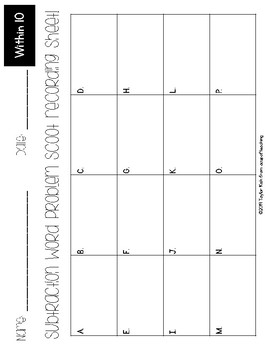# Addition and Subtraction Word Problems Within 10 ScootsSubject
Resource Type
Format
PDF (6 MB|17 pages)
Standards
\$2.00
• Product Description
• Standards

Looking for a fun, engaging way to have your kiddos practice their word problem skills? Check out these addition and subtraction scoots!

This activity set includes 3 scoots:

• Addition Word Problems within 10
• Subtraction Word Problems within 10
• Addition and Subtraction Word Problems within 10

To use, simply choose the scoot you would like to use and print out the task cards on cardstock or laminate them. Then, scatter them throughout your room (or hallway!) and give each of your students a recording sheet. Your students will find the letter on each task card and match it to the box on their recording sheet to write in the correct answer. They'll "scoot" around the room looking for all of the task cards!

Happy Teaching!

Taylor

to see state-specific standards (only available in the US).
Fluently add and subtract within 20 using mental strategies. By end of Grade 2, know from memory all sums of two one-digit numbers.
Add and subtract within 20, demonstrating fluency for addition and subtraction within 10. Use strategies such as counting on; making ten (e.g., 8 + 6 = 8 + 2 + 4 = 10 + 4 = 14); decomposing a number leading to a ten (e.g., 13 - 4 = 13 - 3 - 1 = 10 - 1 = 9); using the relationship between addition and subtraction (e.g., knowing that 8 + 4 = 12, one knows 12 - 8 = 4); and creating equivalent but easier or known sums (e.g., adding 6 + 7 by creating the known equivalent 6 + 6 + 1 = 12 + 1 = 13).
Use addition and subtraction within 20 to solve word problems involving situations of adding to, taking from, putting together, taking apart, and comparing, with unknowns in all positions, e.g., by using objects, drawings, and equations with a symbol for the unknown number to represent the problem.
Fluently add and subtract within 5.
Solve addition and subtraction word problems, and add and subtract within 10, e.g., by using objects or drawings to represent the problem.
Total Pages
17 pages
Included
Teaching Duration
N/A
Report this Resource to TpT
Reported resources will be reviewed by our team. Report this resource to let us know if this resource violates TpT’s content guidelines.
• Ratings & Reviews
• Q & A

Teachers Pay Teachers is an online marketplace where teachers buy and sell original educational materials.